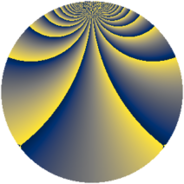# Properties

 Label 1840.2.xLevel $1840$ Weight $2$ Character orbit 1840.x Rep. character $\chi_{1840}(461,\cdot)$ Character field $\Q(\zeta_{4})$ Dimension $352$ Sturm bound $576$

# Related objects

## Defining parameters

 Level: $$N$$ $$=$$ $$1840 = 2^{4} \cdot 5 \cdot 23$$ Weight: $$k$$ $$=$$ $$2$$ Character orbit: $$[\chi]$$ $$=$$ 1840.x (of order $$4$$ and degree $$2$$) Character conductor: $$\operatorname{cond}(\chi)$$ $$=$$ $$16$$ Character field: $$\Q(i)$$ Sturm bound: $$576$$

## Dimensions

The following table gives the dimensions of various subspaces of $$M_{2}(1840, [\chi])$$.

Total New Old
Modular forms 584 352 232
Cusp forms 568 352 216
Eisenstein series 16 0 16

## Trace form

 $$352q + 12q^{6} + O(q^{10})$$ $$352q + 12q^{6} + 12q^{12} - 16q^{14} + 40q^{18} - 16q^{20} + 48q^{22} - 8q^{24} + 32q^{26} - 24q^{27} + 48q^{28} - 40q^{34} - 12q^{36} - 40q^{42} - 72q^{44} + 80q^{47} - 352q^{49} + 80q^{51} - 48q^{52} - 32q^{54} + 56q^{56} + 20q^{58} - 8q^{59} + 56q^{60} + 76q^{62} - 12q^{64} - 80q^{66} + 16q^{70} - 36q^{72} + 40q^{74} - 60q^{78} - 32q^{80} - 352q^{81} + 116q^{82} - 80q^{83} + 32q^{84} + 16q^{86} - 40q^{88} - 72q^{90} - 64q^{91} + 48q^{93} - 48q^{94} - 64q^{95} + 120q^{96} - 56q^{98} - 64q^{99} + O(q^{100})$$

## Decomposition of $$S_{2}^{\mathrm{new}}(1840, [\chi])$$ into newform subspaces

The newforms in this space have not yet been added to the LMFDB.

## Decomposition of $$S_{2}^{\mathrm{old}}(1840, [\chi])$$ into lower level spaces

$$S_{2}^{\mathrm{old}}(1840, [\chi]) \cong$$ $$S_{2}^{\mathrm{new}}(16, [\chi])$$$$^{\oplus 4}$$$$\oplus$$$$S_{2}^{\mathrm{new}}(80, [\chi])$$$$^{\oplus 2}$$$$\oplus$$$$S_{2}^{\mathrm{new}}(368, [\chi])$$$$^{\oplus 2}$$